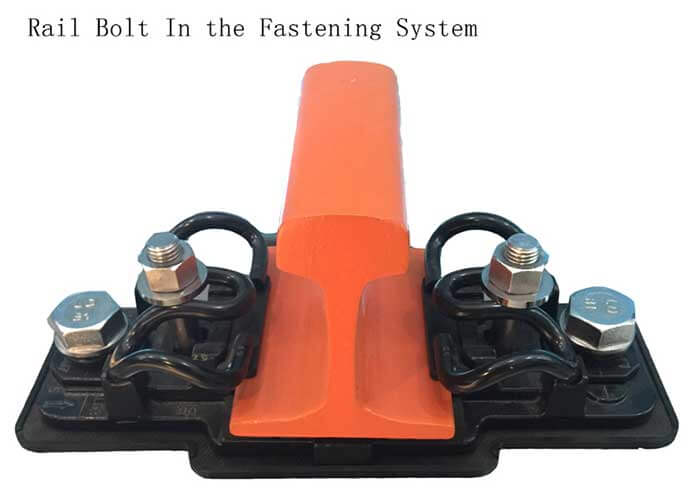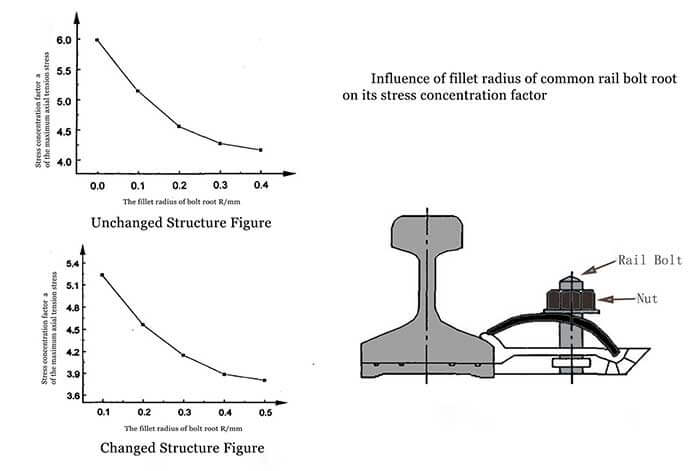# How to Improve Rail Bolt Fatigue Strength in the Fastening System

## Why we need to improve rail bolt fatigue strength in the fastening system?

The arc radius of rail bolt root has a great influence on its stress concentration.Through alleviating its stress concentration and improving the stress distribution, we can effectively increase fatigue strength of rail bolt. Now let’s have related analysis to make an attempt at find an effective method of improving the rail bolt fatigue strength in the fastening system.## 1. Finite element connection model of rail bolt

Rail bolts are connected by rail, rail bolt, nut, cushion and so on. Take the following rail bolt for example, its material is 20MnTiB, its elastic modulus is 210GPa, Poisson's ratio is 0.28, tensile strength is 1040MPa, the yield strength is 940MPa. The bolt length is 72mm, nominal diameter is 24mm, the pitch is 3mm, diameter of thread is 22.051mm, nut diameter is 40mm and rotation length is 27mm.

To analyze the influences of the arc radius of rail bolt root on the maximum axial tension stress and stress concentration factor of the bolt, and reduce calculation workload, rail bolt and its nut can be separately used as different research objects and replace the interaction of rail and nut with the contact load in the premise of keeping the accuracy.

According to the connection structure of rail bolt and its stress characteristic (axial symmetry), the finite element model is established. In addition, because the bolt is in contact with the nut each other, the nonlinear contact analysis should be carried out, but they can’t be considered as the same object for finite element analysis.

The unit division of the finite element model not only affects the calculation speed, but also does the calculation accuracy. Therefore, the unit division is one of the key points in the finite element analysis.Dividing the unit needs to reach the state of reasonable density and stress distribution. In the place of thread where variation of stress is greater, in order to better reflect the changes of stress, and we here should require the use of relatively dense grid. Conversely, other places should be divided into relative sparse grid. Here for our analysis, we adopt quadrilateral axisymmetric plane element.

## 2. Influence of fillet radius of common rail bolt root on its stress concentration factor## 3. Methods of improving the fatigue strength of rail bolts

Maximum fillet radius of rail bolt root thread is determined by the thread geometry, which can’t be arbitrarily increased. In order to increase its fillet radius and improve its fatigue strength, so we must change structures of the bolt and nut. But due to a huge amount of use for rail bolts and nuts, referring to changing their structures, we have to consider higher cost of manufacture and construction.

### 3.1 To change minor radius of rail bolt

The maximum radius of common triangular bottom thread profile is 0.14433753375P, in the premise of keeping basic thread profile unchanged, if we want to further increase maximum radius ,we can be accomplished through minor radius of the fixed bolt, that is, to increase minor radius of outside thread.

### 3.2 Thread profiles of both bolt and nut adopt the circular arc shape

Under the premise of nut thread profile’ keeping unchanged, fillet radius increase of thread profile of bolt root is still limited. To further reduce the root stress concentration and improve fatigue strength of rail bolt, we can make it by changing the structure of bolt connection, such as the use of tension nut, slotted nut and internal oblique nut, to change the thread load distribution. But changes in the structure will cause manufacturing cost to rise up, and the consumption of rail bolt and nut is numerous, so this method is seldom adopted.

After cutting increases in depth at the position of minor radius of the root, the fillet radius also increases. If thread profile of its nut is changed simultaneously and matched with the bolt that is also changed into circular arc shape, that will further increase the fillet radius, but the manufacturing cost of the nut doesn’t increase, too. According to that, the maximum radius should be Rmax=5Pcos30°/16 =0.2706329P. When the pitch P=3mm, Rmax=0.8113 8988mm.

With the increase of minor radius of the bolt, the fillet radius of the bolt root is obviously increased. According to that radius, the stress concentration factor is calculated to be 3.14, and the difference is 0.67, compared with the increase of the bolt (R = 0.5 mm), the relative reduction is about 17.59%. The stress concentration factor is obviously reduced, and the stress concentration in the root of the bolt root is relieved significantly.

Considering that the stress concentration factor of common bolt is R=5.98mm, and the stress concentration factor is 3.14 when changing the thread profiles of the bolt and nut simultaneously, the difference between two factors is 2.84, and relative reduction is 47.49%. So the change of the structure has greatly improved the fatigue strength and service life of rail bolt.

## 4. Conclusion

A. The maximum axial tensile stress and the stress concentration factor of the common rail bolt are obviously decreased with increase of the fillet radius of bolt thread.

B. There are two methods of Increase of fillet radius of bolt root: one is to increase Leveling Height of the thread; the other is to change the structure of the bolt and nut. Through the structure change of the bolt and nut we can get larger fillet radius of bolt root, which is conducive to reducing the influence on the maximum axial tensile stress and stress concentration factor of common rail bolt.

C. The structure change of the bolt and nut can obviously reduce the stress concentration and improve rail bolt fatigue strength, but we should take into account manufacturing cost and manufacture problem.

D. In order to improve the accuracy of stress analysis of rail bolt connection, the finite element analysis method should be adopted, but the bolt and nut can’t be regarded as a whole.

Get in touch